# Power Dissipation, Motor Sizing and Servo Loop Bandwidth

This article discusses how servo loop bandwidth, motor sizing, and power dissipation are all connected. Some background concerning servo loop gains and bandwidths will be provided to facilitate a discussion of two case studies. Case 1 is a move and settle application where it will be proved that servo bandwidth is not related to motor sizing. Case 2 is a disturbance tracking application where servo bandwidth directly impacts motor sizing. In each case, power dissipation is eventually the main driver for sizing a motor.

## Loop Gain, Stability, Bandwidth and Motor Sizing

Servo bandwidth is a direct function of loop gain, which includes gains from the plant, the motor, the sensor, the motor drive, and the control gain. Higher loop gain always results in higher bandwidth. Loop gain can be decreased or increased just by modifying the gain of any one component in the loop (control, sensor, plant, motor or motor drive). This means that there is not a single solution of each gain that results in a targeted bandwidth, and instead, each gain can be lower or higher, as long as the total gain is conserved.

For instance, the sensor gain for system A could be 1 count for 1 µm, and the sensor gain for system B could be 10 counts for 1 µm. System A and B can have the same bandwidth as long as one of the other gains compensates for the 10x difference. In this example, the most probable gain to regulate would be the control gain, system B being 10x less.

Power dissipation aside, loop gain (and resulting bandwidth) cannot grow without bound, rather it is restricted by stability. Stability is stimulated by phase margin at the bandwidth frequency and gain margin when the phase is at -180°.

Higher bandwidths mean the motor will be commanded by the control system to react at higher frequencies. Higher frequencies result in higher required motor forces and higher accelerations, all resulting in higher dissipated power. Dissipated power (or allowable temperature rise), can limit the allowable bandwidth, meaning that although stability might allow a system to have a 100 Hz bandwidth, the dissipated power requirement and motor Km selected might limit the bandwidth to be only 50 Hz. Or conversely, if 100 Hz is required, the motor Km will be sized accordingly to match the power dissipation requirement.

## Case 1- Move and Settle

In this case, it will be illustrated how motor sizing is not coupled to servo bandwidth, but is couple to power dissipated in the motor.

Figure 1 shows a generic block diagram that is valid for nearly all closed-loop control systems. As stated previously, the loop gain is comprised of the control gain (Kcntrl), the plant gain (Kplant), the motor gain (Kt), the drive gain (Kdrive), and the sensor gain (Ksens). Kcntrl, Kdrive, and Kplant are extremely frequency dependent. However, Kt and Ksens are usually constant (up to a few hundred Hz). If a targeted bandwidth frequency is available, one can solve for Kcntrl at preferred bandwidth frequency and verify stability.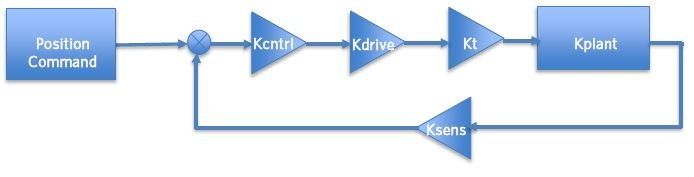Figure 1. System Block Diagram.

In the system illustrated in Figure 1, the plant is usually a fixed entity. For the sake of this example, let’s assume the sensor gain is also fixed. Now let’s assume system A had a motor gain of 10 N-m/amp and system B, 1 Nm/amp. As described earlier, system A and B can both have the same bandwidth, provided the total loop gain is the same. In this instance, if Kcntrl system B were increased by 10x, system A and system B would have the same loop gain, and thus the same servo bandwidth.

This example is intended to show that there is not a unique motor gain that provides a preferred servo bandwidth. There is, however, a unique torque needed to move the load, given the inertia of the load and its acceleration. Changing the motor gain (N-m/amp) between system A and B means that to conserve the torque developed by the motors, the current flowing via the motor for system B is 10x larger than that of system A. This also means that the dissipated power of system B will be larger than that of system A, and that system B will have a higher coil temperature.

When moving a load from one location to another, the servo bandwidth does not greatly impact the motion trajectory. Figure 2 below shows how different servo bandwidths will develop very similar motion trajectories. Noticeably the 100 Hz servo follows the commanded position better than the 50 Hz, but the dominant acceleration is formed from the trajectory itself.

The acceleration, together with the duty cycle of the movement, and the mass or inertia of the load, all impact the dissipated power (Pd).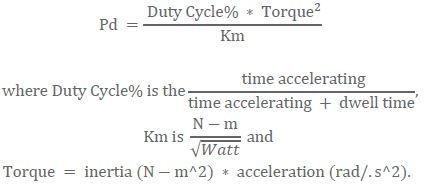Knowing an allowable Pd means one can solve for a Km value and motor sizing is currently complete. Motor current and voltage can be traded by the motor design team to arrive at the optimal Kt, based on the available bus voltage.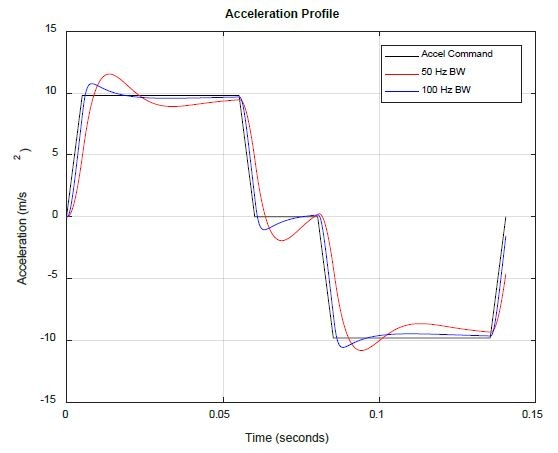Figure 2. Motion Trajectory (Black - Commanded, Red - 50 Hz, Green - 100 Hz).

## Case 2 – Disturbance Tracking

In cases where the control system is reacting to broadband energy (trying to hold position, focus, or velocity), the servo bandwidth may be restricted by motor constant Km. As the units of the motor constant state, Km is a measure of a motor’s ability to create torque per the square root of dissipated power.

Figure 3 shows a system where the job of the control system is to reduce focus error between the optical structure and M2, while M1 is exposed to a disturbance force, forming motion Z1(t). In the example, the input spectrum Z1 (t) has broadband energy up to the kHz frequency range.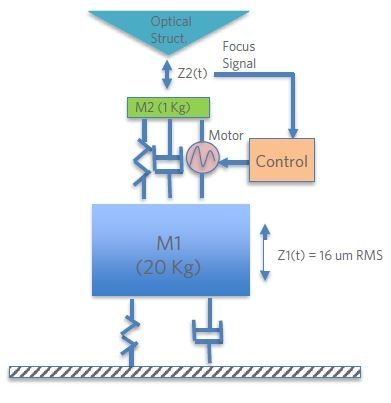Figure 3. Simple Disturbance Tracking System.

Figure 4 illustrates the plant and loop transfer operations for the system. The loop is displaying ~50 Hz 0 dB crossing. This frequency is called the “servo bandwidth”. Figure 5 is a plot of motor force for the 50 Hz case and also the 100 Hz 0 dB case, illustrating how the bandwidth significantly affects the amplitude of the motor force signal. Dissipated power in the system is;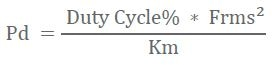where Km is the motor constant in, and Duty Cycle is 100%. Km is calculated using;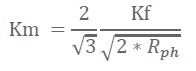In their example, Km is calculated to be 29 N/sqrt (W, where Kf = 50 N/amp, and Rphase = 2 ohms. The dissipated power for these two cases is 12 watts for the 50 Hz case and 75 watts for the 100 Hz case. Evidently, the higher bandwidth case requires more power (6x increase) to monitor the higher frequencies.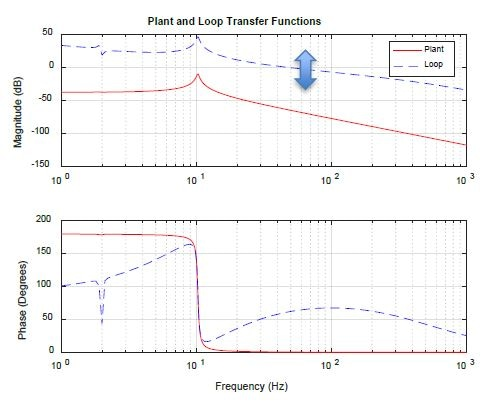Figure 4. Plant and Loop Transfer Functions.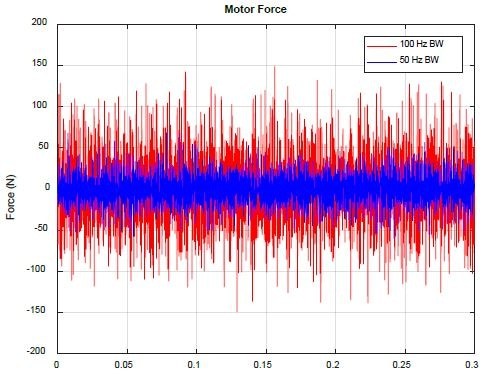Figure 5. Motor force for 50 Hz vs. 100 Hz Bandwidth.

The example illustrated is summarized in Figure 6. The net result is that higher bandwidth systems monitor higher frequency disturbances. Higher frequency disturbances normally mean higher accelerations and thereby higher required motor forces. Power dissipation goes with the square of motor force, so any increase in bandwidth frequency can have a major impact on motor dissipated power and motor sizing.

In Figure 6, the final plot is the resulting acceleration for three cases of servo bandwidths. The yellow is for the 100 Hz case, green for the 75 case, and red for the 50 Hz case. As the pictorials illustrate, the RMS of acceleration, and resulting force, is the area under the acceleration spectrum plot, and the smaller the bandwidth is, the smaller the RMS acceleration will be.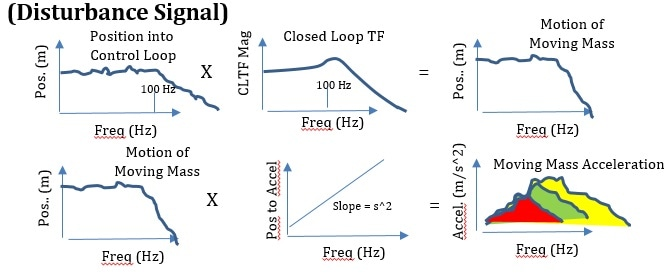Figure 6. Pictorial of Analysis.

## Case Study Summaries

Described here are two very typical, yet different, cases that impact motor sizing. Case 1 is when the control system has to transfer a load from position A to position B and repeat. Case 2 is where the control system has to monitor (or reject) a disturbance signal. In each case, power dissipated in the motor is the ultimate driving factor.

In case 1, the dissipated power is mainly a function of the duty cycle of motion, the moving mass (or inertia), and the acceleration reached during the move and is autonomous of servo loop bandwidth. In this case, the “backbone” of the motion trajectory is the same autonomously of servo bandwidth (within the sum of servo tracking error).

In case 2, however, dissipated power is mostly driven by the servo bandwidth. Higher bandwidth servos ask the controller to monitor higher frequencies, which means higher accelerations and higher motor forces. In the example, a 2x increase in servo bandwidth developed 6x more dissipated power in the motor.

In both scenarios, servo bandwidth is eventually governed by servo stability, meaning gain margin and phase margin must always be fulfilled. In certain cases like case 2 described here, the servo bandwidth might not be restricted by stability, but by allowable dissipated power.

In both cases, dissipated power is applied to size the motor. The load mass (or inertia), its acceleration, and its duty cycle all integrate to influence motor power dissipation.

## Conclusions

Motor sizing is possible by allowable power dissipation in the motor. When selecting a motor, one has to consider how it will be used and do the suitable analysis to arrive at the expected motor power dissipation. Explained here are two cases that need two different types of analysis. In case 1, the analysis is concentrated on the motion trajectory, while in case 2, the analysis is concentrated on the servo bandwidth. In both scenarios, the acceleration of the load, and its mass drive motor power dissipation.This information has been sourced, reviewed and adapted from materials provided by Celera Motion.

## Citations

• APA

Celera Motion. (2019, April 19). Power Dissipation, Motor Sizing and Servo Loop Bandwidth. AZoSensors. Retrieved on May 26, 2020 from https://www.azosensors.com/article.aspx?ArticleID=1021.

• MLA

Celera Motion. "Power Dissipation, Motor Sizing and Servo Loop Bandwidth". AZoSensors. 26 May 2020. <https://www.azosensors.com/article.aspx?ArticleID=1021>.

• Chicago

Celera Motion. "Power Dissipation, Motor Sizing and Servo Loop Bandwidth". AZoSensors. https://www.azosensors.com/article.aspx?ArticleID=1021. (accessed May 26, 2020).

• Harvard

Celera Motion. 2019. Power Dissipation, Motor Sizing and Servo Loop Bandwidth. AZoSensors, viewed 26 May 2020, https://www.azosensors.com/article.aspx?ArticleID=1021.